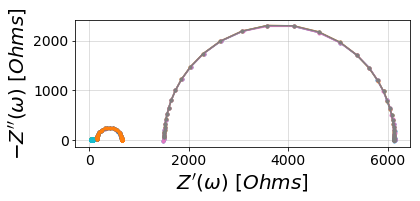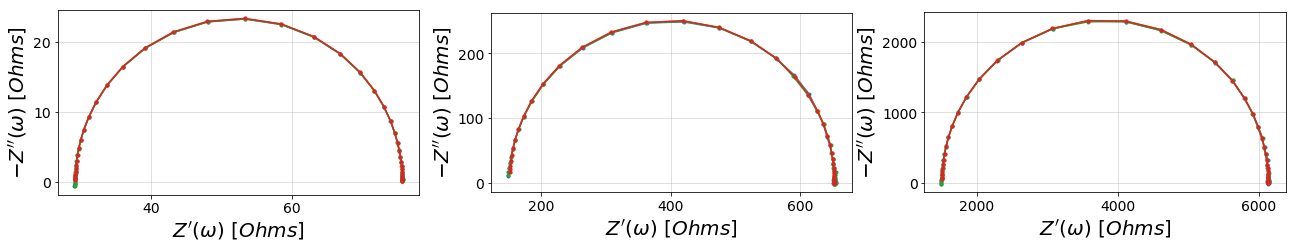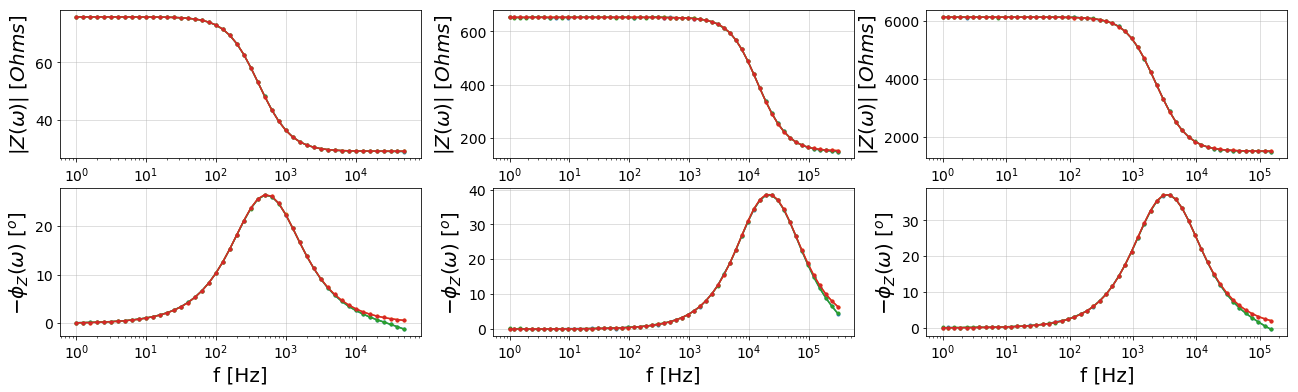# Looping through and fitting multiple impedance data sets¶

:

import glob
import numpy as np
import os


## 1. Find all files that match a specified pattern¶

### Using a search string to find .z files that contain “Circuit” at the beginning and EIS towards the end¶

:

directory = r'../../../data/'
all_files = glob.glob(os.path.join(directory, 'Circuit*EIS*.z'))
all_files

:

['../../../data/Circuit3_EIS_1.z',
'../../../data/Circuit1_EIS_2.z',
'../../../data/Circuit2_EIS_1.z',
'../../../data/Circuit3_EIS_2.z',
'../../../data/Circuit1_EIS_1.z',
'../../../data/Circuit2_EIS_2.z']


#### 2. Use preprocessing module to read in ZPlot data¶

:

from impedance import preprocessing
# Initialize some empty lists for the frequencies and Z data
freqs = []
Zs = []

# Now loop through file names in our list and extract data one by one
for filename in all_files:
freqs.append(f)
Zs.append(Z)

# Check to see if we extracted data for all the files
print(np.shape(Zs), np.shape(all_files))

(6,) (6,)


## 3. Create a list of circuit models¶

:

from impedance.models.circuits import CustomCircuit
# This data comes from dummy circuits I made to check measurement bias in
# our potentiostat, so I know a priori its an R-RC circuit

circuits = []

circ_string = 'R0-p(R1,C1)'
initial_guess = [100, 400, 1e-5]

# Now loop through data list to create circuits and fit them
for f, Z, filename in zip(freqs, Zs, all_files):
name = filename.split('/')[-1]
circuit = CustomCircuit(circ_string, initial_guess=initial_guess, name=name)
circuit.fit(f, Z)
circuits.append(circuit)


We now have a list of our circuit class objects, all fit to different sets of data. As you may notice from the file names, there are three unique circuits each with a replicate set of data. We expect each of the replicates to fit similarly.

:

for circuit in circuits:
print(circuit)


Name: Circuit3_EIS_1.z
Circuit string: R0-p(R1,C1)
Fit: True

Initial guesses:
R0 = 1.00e+02 [Ohm]
R1 = 4.00e+02 [Ohm]
C1 = 1.00e-05 [F]

Fit parameters:
R0 = 1.51e+03  (+/- 2.62e+00) [Ohm]
R1 = 4.63e+03  (+/- 3.14e+00) [Ohm]
C1 = 2.02e-08  (+/- 5.39e-11) [F]

Name: Circuit1_EIS_2.z
Circuit string: R0-p(R1,C1)
Fit: True

Initial guesses:
R0 = 1.00e+02 [Ohm]
R1 = 4.00e+02 [Ohm]
C1 = 1.00e-05 [F]

Fit parameters:
R0 = 2.91e+01  (+/- 3.58e-02) [Ohm]
R1 = 4.67e+01  (+/- 4.64e-02) [Ohm]
C1 = 1.04e-05  (+/- 2.91e-08) [F]

Name: Circuit2_EIS_1.z
Circuit string: R0-p(R1,C1)
Fit: True

Initial guesses:
R0 = 1.00e+02 [Ohm]
R1 = 4.00e+02 [Ohm]
C1 = 1.00e-05 [F]

Fit parameters:
R0 = 1.50e+02  (+/- 3.23e-01) [Ohm]
R1 = 5.02e+02  (+/- 3.57e-01) [Ohm]
C1 = 3.12e-08  (+/- 7.79e-11) [F]

Name: Circuit3_EIS_2.z
Circuit string: R0-p(R1,C1)
Fit: True

Initial guesses:
R0 = 1.00e+02 [Ohm]
R1 = 4.00e+02 [Ohm]
C1 = 1.00e-05 [F]

Fit parameters:
R0 = 1.51e+03  (+/- 2.68e+00) [Ohm]
R1 = 4.63e+03  (+/- 3.21e+00) [Ohm]
C1 = 2.02e-08  (+/- 5.52e-11) [F]

Name: Circuit1_EIS_1.z
Circuit string: R0-p(R1,C1)
Fit: True

Initial guesses:
R0 = 1.00e+02 [Ohm]
R1 = 4.00e+02 [Ohm]
C1 = 1.00e-05 [F]

Fit parameters:
R0 = 2.91e+01  (+/- 3.63e-02) [Ohm]
R1 = 4.67e+01  (+/- 4.69e-02) [Ohm]
C1 = 1.04e-05  (+/- 2.95e-08) [F]

Name: Circuit2_EIS_2.z
Circuit string: R0-p(R1,C1)
Fit: True

Initial guesses:
R0 = 1.00e+02 [Ohm]
R1 = 4.00e+02 [Ohm]
C1 = 1.00e-05 [F]

Fit parameters:
R0 = 1.50e+02  (+/- 3.19e-01) [Ohm]
R1 = 5.02e+02  (+/- 3.53e-01) [Ohm]
C1 = 3.12e-08  (+/- 7.70e-11) [F]



Now we’ll get the impedance predicted by the fit parameters

:

fits = []
for f, circuit in zip(freqs, circuits):
fits.append(circuit.predict(f))


## 4. Plot the data and fits¶

Now we can visualize the data and fits. For now we’ll place them all on the same axis

:

import matplotlib.pyplot as plt
from impedance.visualization import plot_nyquist, plot_bode

:

fig, ax = plt.subplots()

for fit, Z in zip(fits, Zs):
# Plotting data
plot_nyquist(ax, Z)

# Plotting fit
plot_nyquist(ax, fit)

plt.show()Since the circuits have different orders of magnitude impedance, this looks bad so let’s put each pair of data on separate axes.

:

# Nyquist plots
fig, axes = plt.subplots(ncols=3, figsize=(22,6))
for circuit, Z, fit in zip(circuits, Zs, fits):
n = int(circuit.name.split('Circuit')[-1].split('_'))
plot_nyquist(axes[n - 1], Z)
plot_nyquist(axes[n - 1], fit)

# Bode plots
fig, axes = plt.subplots(nrows=2, ncols=3, figsize=(22,6))
for circuit, f, Z, fit in zip(circuits, freqs, Zs, fits):
n = int(circuit.name.split('Circuit')[-1].split('_'))
plot_bode([axes[n - 1], axes[n - 1]], f, Z)
plot_bode([axes[n - 1], axes[n - 1]], f, fit)

plt.show()